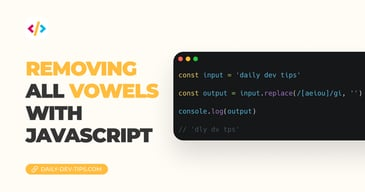Subscribe

# What is the difference between two times? ⌚️

✍️

Calculate the difference between two times in JavaScript

27 Aug, 2020 · 4 min read

📣 Chris, make a speedtest report of this function! Uhh, Ok! No problem but shit, my math is not good.

...

Opens up Time diff website -> Pastes two times -> reports back.

It might be just my math being so bad, but let's make a simple real-world tool that will give us the time past two times.

It will end up looking like this Codepen.

See the Pen What is the difference between the two times? ⌚️ by Chris Bongers (@rebelchris) on CodePen.

``````<div class="container">
<div class="dates">
<div class="start">
<i>Start time:</i> <br />
<input type="number" min="0" max="24" id="s_h" placeholder="HH" />
<input type="number" min="0" max="60" id="s_m" placeholder="MM" />
<input type="number" min="0" max="60" id="s_s" placeholder="SS" />
</div>
<div class="space"></div>
<div class="end">
<i>End time:</i> <br />
<input type="number" min="0" max="24" id="e_h" placeholder="HH" />
<input type="number" min="0" max="60" id="e_m" placeholder="MM" />
<input type="number" min="0" max="60" id="e_s" placeholder="SS" />
</div>
</div>
<button id="button">Perform some magic ✨</button>
<div id="output"></div>
</div>``````

We need two divs to keep three inputs for the hours, minutes, and seconds. Then we need a button to perform our magic on click and an output div to place the result in!

Let's make the above show a bit nicer:

``````.container {
display: flex;
justify-content: center;
align-items: center;
flex-direction: column;
height: 100vh;
background: #4ea8de;
}
.dates {
display: flex;
text-align: center;
}
.dates i {
margin-bottom: 10px;
}
.dates input {
width: 40px;
margin-top: 10px;
}
.space {
margin: 0px 10px;
}
button {
margin-top: 20px;
font-size: 24px;
background: #7400b8;
color: #80ffdb;
cursor: pointer;
transition: all 0.5s ease;
}
button:hover {
background: #6930c3;
}
#output {
margin-top: 20px;
font-size: 18px;
}``````

I'm mainly using flexbox to center some elements, adding some margins and colors to make it look better.

Note that we are adding a transition to the button; this will make the button background quickly fade instead of a complex hover effect. Another cool transition is this Hamburger menu.

## JavaScript time passed between two times permalink

On to the magic part, `JavaScript` is what is going to make everything work.

First, we need to define all our variables.

• The six inputs
• The button
• and the output div
``````const startHour = document.getElementById('s_h'),
startMinute = document.getElementById('s_m'),
startSecond = document.getElementById('s_s'),
endHour = document.getElementById('e_h'),
endMinute = document.getElementById('e_m'),
endSecond = document.getElementById('e_s'),
button = document.getElementById('button'),
output = document.getElementById('output');``````

As you can see above, we can add one row of variables. It just beats writing const or var every time. You can space them out with commas.

Now let's add a click event to our button:

``````button.addEventListener('click', function () {
// Code coming here
// 👇
});``````

Awesome, now we need to get our start and end date inside this click:

``````let startDate = new Date(
2020,
05,
05,
startHour.value,
startMinute.value,
startSecond.value
);
let endDate = new Date(
2020,
05,
05,
endHour.value,
endMinute.value,
endSecond.value
);``````

We define a random day. We are only using the time settings in this example.

Now let's get the difference between these two times!

``let difference = endDate.getTime() - startDate.getTime();``

The `getTime()` function returns the timestamp, which makes it easier to calculate with

Let's first check if the end date is not in the future:

``````if (difference < 0) {
output.innerHTML = 'Wow dude, you just went back to the future! 👽';
} else {
// Code coming below
// 👇
}``````

So we are giving the user feedback if they are trying to scam us, the tug! 👀

``````difference = difference / 1000;
let hourDifference = Math.floor(difference / 3600);
difference -= hourDifference * 3600;
let minuteDifference = Math.floor(difference / 60);
difference -= minuteDifference * 60;
output.innerHTML = `\${hourDifference} hours, \${minuteDifference} minutes, \${difference} seconds`;``````

Wow, hold your horses. What's going on here? 🤠

First, we need to divide the difference by 1000. This removes the milliseconds.

Then we say give us the hours past in the difference, 3600 = (60 seconds * 60 minutes = 1 hour). We are using Math.floor always to round downwards. We don't want 0.9 hours to become 1.

Next, we need to detract the passed hours from the difference.

We do the same for the minutes, but we only need to divide by 60 since we detracted the hours already. Then again, we remove whatever minutes passed.

The difference we end up with is the seconds!

Then we use liquid template tags (`\${variable}`) to return the result to the user!

Voila! We are now masters of time and the universe 🧙‍♂️!

Tweet this tip

### Removing all vowels with JavaScript

3 Dec, 2022 · 2 min read### 10 games to learn JavaScript

2 Dec, 2022 · 3 min readJoin 2103 devs and subscribe to my newsletter

• 1000 articles written
• 2103 devs subscribed
• 529116 words written